# Ordinal Regression using SPSS Statistics

## Introduction

Ordinal logistic regression (often just called 'ordinal regression') is used to predict an ordinal dependent variable given one or more independent variables. It can be considered as either a generalisation of multiple linear regression or as a generalisation of binomial logistic regression, but this guide will concentrate on the latter. As with other types of regression, ordinal regression can also use interactions between independent variables to predict the dependent variable.

For example, you could use ordinal regression to predict the belief that "tax is too high" (your ordinal dependent variable, measured on a 4-point Likert item from "Strongly Disagree" to "Strongly Agree"), based on two independent variables: "age" and "income". Alternately, you could use ordinal regression to determine whether a number of independent variables, such as "age", "gender", "level of physical activity" (amongst others), predict the ordinal dependent variable, "obesity", where obesity is measured using using three ordered categories: "normal", "overweight" and "obese".

Having carried out ordinal regression, you will be able to determine which of your independent variables (if any) have a statistically significant effect on your dependent variable. For categorical independent variables (e.g., "Political party last voted for", which in Great Britain, has 3 groups for this example: "Conservatives", "Labour" and "Liberal Democrats"), you will be able to interpret the odds that one group (e.g., "Conservative" supporters) had a higher or lower value on your dependent variable (e.g., a higher value could be stating that they "Strongly agree" that "Tax is too high" rather than stating that they "Disagree") compared to the second group (e.g., "Labour" supporters). For continuous independent variables (e.g., "age", measured in years), you will be able to interpret how a single unit increase or decrease in that variable (e.g., a one year increase or decrease in age), was associated with the odds of your dependent variable having a higher or lower value (e.g., a one year increase in participants' age increasing the odds that they would consider tax to be too high). You will also be able to determine how well your ordinal regression model predicts the dependent variable.

This "quick start" guide shows you how to carry out ordinal regression using SPSS Statistics and explain what you need to interpret and report. However, before we introduce you to this procedure, you need to understand the different assumptions that your data must meet in order for ordinal regression to give you a valid result. We discuss these assumptions next.

## Assumptions

When you choose to analyse your data using ordinal regression, part of the process involves checking to make sure that the data you want to analyse can actually be analysed using ordinal regression. You need to do this because it is only appropriate to use ordinal regression if your data "passes" four assumptions that are required for ordinal regression to give you a valid result. In practice, checking for these four assumptions just adds a little bit more time to your analysis, requiring you to click a few more buttons in SPSS Statistics when performing your analysis, as well as think a little bit more about your data, but it is not a difficult task.

Before we introduce you to these four assumptions, do not be surprised if, when analysing your own data using SPSS Statistics, one or more of these assumptions is violated (i.e., not met). This is not uncommon when working with real-world data rather than textbook examples, which often only show you how to carry out ordinal regression when everything goes well! However, don’t worry. Even when your data fails certain assumptions, there is often a solution to overcome this. First, let's take a look at these four assumptions:

• Assumption #1: Your dependent variable should be measured at the ordinal level. Examples of ordinal variables include Likert items (e.g., a 7-point scale from "strongly agree" through to "strongly disagree"), amongst other ways of ranking categories (e.g., a 3-point scale explaining how much a customer liked a product, ranging from "Not very much", to "It is OK", to "Yes, a lot"). You can learn more about ordinal variables in our article: Types of Variable.
• Assumption #2: One or more independent variables that are continuous, ordinal or categorical (including dichotomous variables). However, ordinal independent variables must be treated as being either continuous or categorical. They cannot be treated as ordinal variables when running an ordinal logistic regression in SPSS Statistics; something we highlight later in the guide. Examples of continuous variables that meet this criterion include age (measured in years), revision time (measured in hours), income (measured in US dollars), intelligence (measured using IQ score), exam performance (measured from 0 to 100), weight (measured in kg), and so forth. Types of ordinal variables are presented in the previous bullet. Example categorical variables include gender (e.g., 2 groups: male and female), ethnicity (e.g., 3 groups: Caucasian, African American and Hispanic), profession (e.g., 5 groups: surgeon, doctor, nurse, dentist, therapist), and so forth. Again, you can learn more about variables in our article: Types of Variable.
• Assumption #3: There is no multicollinearity. Multicollinearity occurs when you have two or more independent variables that are highly correlated with each other. This leads to problems with understanding which variable contributes to the explanation of the dependent variable and technical issues in calculating an ordinal regression. Determining whether there is multicollinearity is an important step in ordinal regression. Unfortunately, testing for this assumption can require creating dummy variables for your categorical variables (i.e., dummy variables are new variables based on the values of your existing data). The number of dummy variables you have to create in SPSS Statistics will depend on how many categorical independent variables you have, and how many groups each of these independent variables has. If you only have one categorical independent variable with just three groups (e.g., the variable, "Ethnicity", with three grous: "Caucasian", "African American" and "Hispanic"), you will only have to create two dummy variables. However, if you have multiple categorical independent variables, each with three or more groups, you will have to create quite a lot of these dummy variables. In our enhanced ordinal regression guide, we show you: (a) how to create these dummy variables using SPSS Statistics; (b) how to test for multicollinearity using SPSS Statistics; (c) some of the things you will need to consider when interpreting your data; and (d) an option to continue with your analysis if your data fails to meet this assumption.
• Assumption #4: You have proportional odds, which is a fundamental assumption of this type of ordinal regression model; that is, the type of ordinal regression that we are using in this guide (i.e., cumulative odds ordinal regression with proportional odds). The assumption of proportional odds means that each independent variable has an identical effect at each cumulative split of the ordinal dependent variable. It is tested in SPSS Statistics using a full likelihood ratio test comparing the fitted location model to a model with varying location parameters. Whilst this can sound a little complicated, we explain it in our enhanced ordinal regression guide. The only problem with this test is that it can flag violations that do not exist, so we also show you how to run separate binomial logistic regressions on cumulative dichotomous dependent variables to further determine if this assumption is met. Again, this sounds complicated, but we show you how to do it using SPSS Statistics in our enhanced ordinal regression guide, as well as explaining how to interpret the results from this test.

You can check assumptions #3 and #4 using SPSS Statistics. Assumptions #1 and #2 should be checked first, before moving onto assumptions #3 and #4. We suggest testing these assumptions in this order because it represents an order where, if a violation to the assumption is not correctable, you will no longer be able to use ordinal regression (although you may be able to run another statistical test on your data instead). Just remember that if you do not run the statistical tests on these assumptions correctly, the results you get when running ordinal regression might not be valid. This is why we dedicate a number of sections of our enhanced ordinal regression guide to help you get this right. You can find out about our enhanced content as a whole here, or more specifically, learn how we help with testing assumptions here.

In the section, Procedure, we illustrate the SPSS Statistics procedure to perform an ordinal regression assuming that no assumptions have been violated. First, we introduce the example that is used in this guide.## Example

Taxes have the ability to elicit strong responses in many people with some thinking they are too high, whilst others think they should be higher. A researcher conducted a simple study where they presented participants with the statement: "Tax is too high in this country", and asked them how much they agreed with this statement. They had four options of how to respond: "Strongly Disagree", "Disagree", "Agree" or "Strongly Agree". These ordered responses were the categories of the dependent variable, tax_too_high. The researcher asked participants a number of simple questions, including whether they owned their own business ( biz_owner), their age (age) and which political party they last voted for (politics). The researcher wishes to know the relationship between the independent variable – biz_owner, age and politics – and the dependent variable, tax_too_high.

Note: For those readers that are not familiar with the British political system, we are taking a sterotypical approach to the three major political parties, whereby the Liberal Democrats and Labour are parties in favour of high taxes and the Conservatives are a party favouring lower taxes.

## Setup in SPSS Statistics

In SPSS Statistics, we created four variables: (1) the dependent variable, tax_too_high, which has four ordered categories: "Strongly Disagree", "Disagree", "Agree" and "Strongly Agree"; (2) the independent variable, biz_owner, which has two categories: "Yes" and "No"; (3) the independent variable, politics, which has three categories: "Con", "Lab" and "Lib" (i.e., to reflect the Conservatives, Labour and Liberal Democrats); and (4) the independent variable, age, which is the age of the participants.

Note: In the SPSS Statistics procedures you are about to run, you need to separate the variables into covariates and factors. For these particular procedures, SPSS Statistics classifies continuous independent variables as covariates and categorical independent variables as factors. Thus, age is considered a covariate and politics and biz_owner are considered factors.

In our enhanced ordinal regression guide, we show you how to correctly enter data in SPSS Statistics to run an ordinal regression when you are also checking for assumptions #3 and #4 (see the Assumptions section). You can learn about our enhanced data setup content here. Alternately, we have a generic, "quick start" guide to show you how to enter data into SPSS Statistics, available here.

## Test Procedure in SPSS Statistics

In SPSS Statistics, an ordinal regression can be carried out using one of two procedures: PLUM and GENLIN. Whilst GENLIN has a number of advantages over PLUM, including being easier and quicker to carry out, it is only available if you have SPSS Statistics' Advanced Module. Therefore, in the procedure sections in this "quick start" guide, we focus on the PLUM command instead (N.B., in our enhanced ordinal regression guide, we also show you how to use the GENLIN procedure).

In addition, there is more than one type of ordinal regression that can be used to analyse ordinal dependent variables. To understand these different types, consider the definition of an ordinal variable as a categorical variable with ordered categories (e.g., the dependent variable, "Tax is too high", with four ordered categories: "1 = Strongly Agree", "2 = Agree", "3 = Disagree" and "4 = Strongly Disagree"; or the dependent variable, "Obesity", with three ordered categories: "1 = Obese", "2 = At risk" and "3 = Healthy"). The critical question is, "How do we represent the order of the categories in our analyses?", since this is something that you have to do when carrying out ordinal regression.

In order to capture the ordered nature of these categories, a number of approaches have been developed, based around the use of cumulative, adjacent or continuation categories. For each of these three approaches, different ordinal regression models have been developed. We show you the most popular type of ordinal regression, known as cumulative odds ordinal logistic regression with proportional odds, which uses cumulative categories. What these terms mean, the relationship of ordinal to binomial logistic regression and the assumption of proportional odds are discussed in our enhanced guide. For the purpose of this "quick start" guide, you can simply think of it as ordinal regression, but if you are writing up your methodology or results section, you should highlight the type of ordinal regression you used.

To carry out ordinal regression in SPSS Statistics, there are five sets of procedures. Whilst this sounds like a lot, they are all fairly straight forward. The only procedures that we do not cover below are those required to test assumptions #3 and #4 of the ordinal regression test, as mentioned earlier (see the Assumptions section). Before we take you through each of these five sets of procedures, we have briefly outlines what they are below:

• Procedure #1 – Working with OMS: One of the major drawbacks of the PLUM procedure, despite being SPSS Statistics' dedicated ordinal regression procedure, is that it does not produce all the statistical output you need; in particular, it does not output "odds ratios" or their "95% confidence intervals" (N.B., we explain more about these statistics later). Instead, it produces "log odds". However, you can instruct SPSS Statistics to convert the differences in log odds into the odds ratios you need. To do this, we show you how to use the Output Management System (OMS) Control Panel. This basically stores the information you need when running Procedure #2 below, so that you can use SPSS Statistics to calculate the odds ratios later (i.e., using Procedures #3, #4 and #5).
• Procedure #2 – Running the PLUM procedure: The PLUM procedure in SPSS Statistics produces some of the main results for your ordinal regression analysis, including predicted probabilities, amongst other useful statistical measures that you will need for later analysis. Some of this will require using syntax, but we explain what you need to do.
• Procedure #3 – Outputting the PLUM parameters estimates using OMS: Once you have run the PLUM procedure, you need to go back to the OMS control panel and get SPSS Statistics to output the file containing the Parameter Estimates table's information that has been stored in memory.
• Procedure #4 – Saving the newly-created file: Assuming that you have followed the procedure above, you will not only have generated the output in the usual way (i.e., in the Output Viewer window), but you will have also created a new SPSS Statistics data file. This file is not automatically saved, so you should save it before proceeding further.
• Procedure #5 – Generating odds ratios: Once you have saved the file, you need to generate the odds ratios and their 95% confidence intervals, which you can do using the saved file from Procedure #4. This requires the use of syntax, but we show you what syntax to copy into the Syntax Editor.

Procedure #1 is presented on this page, whilst Procedures #2, #3 and #4 are on the next page and Procedure #5 on page 3. Just remember that you cannot obtain all the statistics you require to carry out ordinal regression without going through these procedures in order.

## Procedure I – Working with OMS

The following instructions show you how to set up SPSS Statistics to store the information from the Parameter Estimates table into memory, which you will later use to produce "odds ratios" and their "95% confidence intervals" (N.B., we explain more about these statistics later):

Join the 10,000s of students, academics and professionals who rely on Laerd Statistics.
• Click Utilities > OMS Control Panel... on the main menu, as shown below: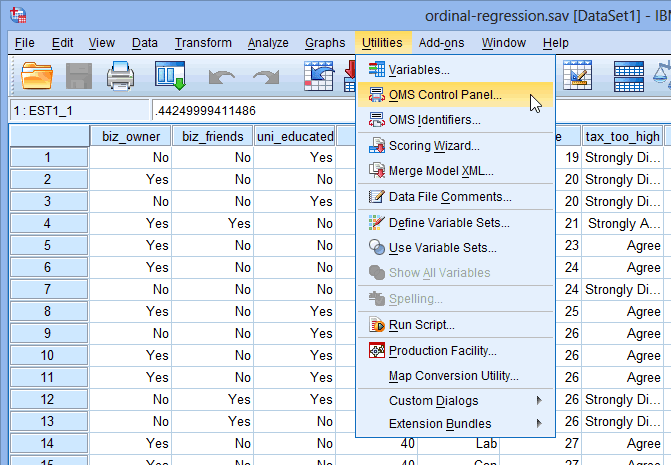Published with written permission from SPSS Statistics, IBM Corporation.

You will be presented you with the Output Management System Control Panel dialogue box, as shown below: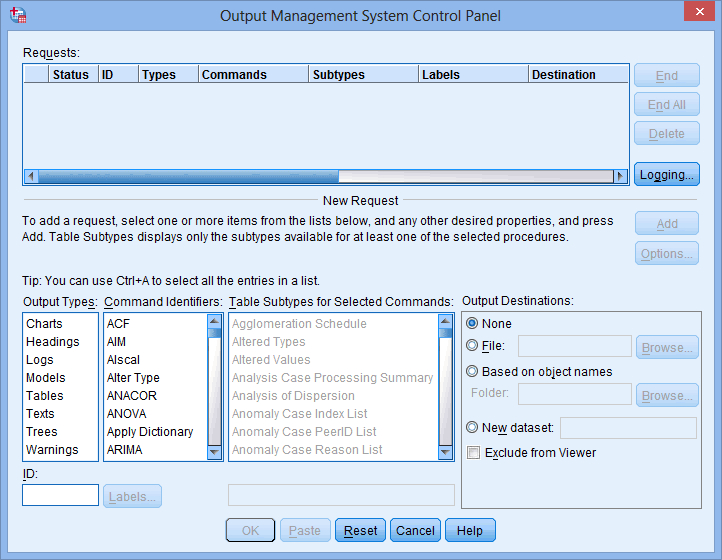Published with written permission from SPSS Statistics, IBM Corporation.

• In this order, select "Tables" in the Output Types: box, "PLUM" in the Command Identifiers: box and "Parameter Estimates" in the
Table Subtypes for Selected Commands: box, as shown below: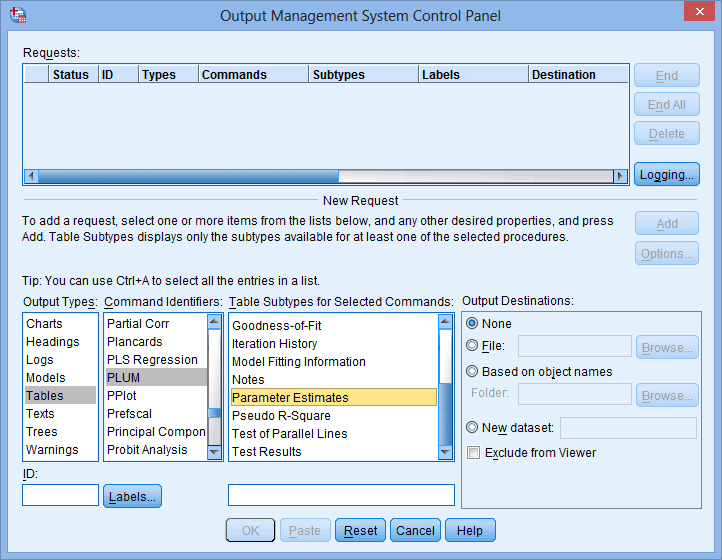Published with written permission from SPSS Statistics, IBM Corporation.

Explanation: You have just instructed SPSS Statistics to 'listen' for when a Parameter Estimates (Table Subtypes for Selected Commands:) table (Output Types:) is produced via the PLUM procedure (Command Identifiers:).

• Select the New dataset: box from the Output Destinations: area and enter "plum" in the box, as shown below: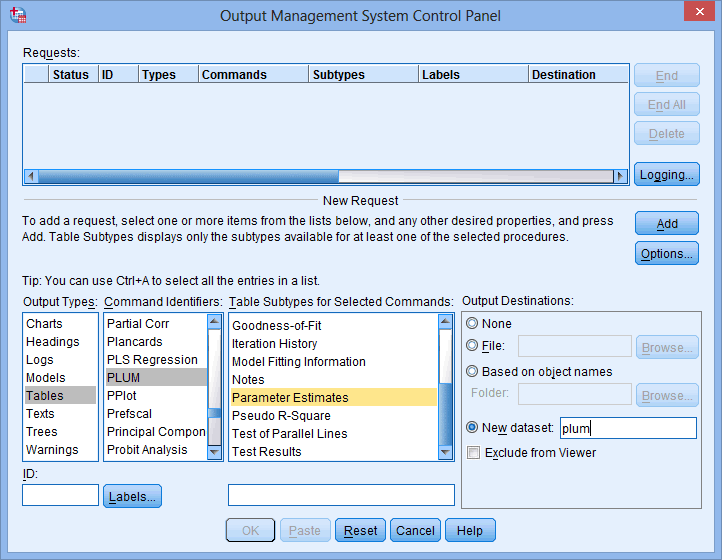Published with written permission from SPSS Statistics, IBM Corporation.

• Click the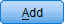button in the –New Request– area. This will add this request to the Requests: box, as shown below: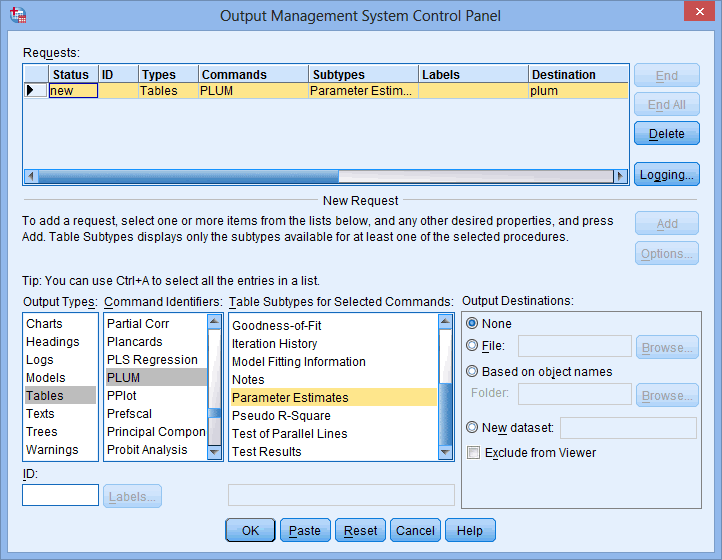Published with written permission from SPSS Statistics, IBM Corporation.

• Click the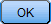button. You will be presented with the OMS Control Panel: Summary dialogue box, as shown below: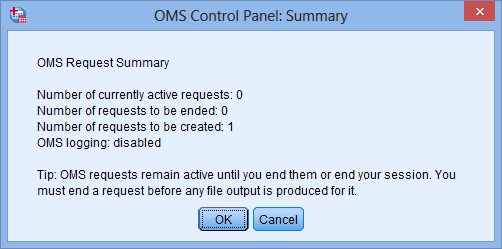Published with written permission from SPSS Statistics, IBM Corporation.

• Click the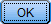button to exit.

Go to the next page to be shown how to run the PLUM procedure in SPSS Statistics.